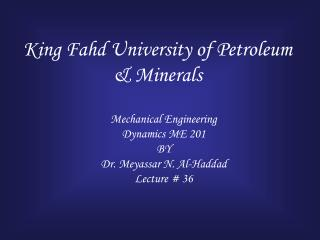# King Fahd University of Petroleum & Minerals - PowerPoint PPT PresentationDownload PresentationKing Fahd University of Petroleum & Minerals

King Fahd University of Petroleum & MineralsDownload Presentation## King Fahd University of Petroleum & Minerals

- - - - - - - - - - - - - - - - - - - - - - - - - - - E N D - - - - - - - - - - - - - - - - - - - - - - - - - - -
##### Presentation Transcript

1. King Fahd University of Petroleum & Minerals Mechanical Engineering Dynamics ME 201 BY Dr. Meyassar N. Al-Haddad Lecture # 36

2. Chapter 18Planar Kinetics of Rigid Bodies:Work and Energy

3. Kinetic Energy

4. General Translation Rotation

5. Rotation

6. General Plane Motion (Translation and Rotation)

7. Example Total K.E. ? K.E. of the Body of Bike and man ( translational ) K.E of the wheel ( Translational + Rotational ) Total K.E. = 2 Twheel +T B+Man

8. mB=6 kg mD=10 kg mC=12 kg No slipping Total K.E = ? Example 18-1 Block (Translation) Disk (Rotation) Cylinder ( Translation &Rotation)

9. Problem wAB=wCD=10 Ib wBC=20 Ib Total K.E = ?

10. The work of a force

11. Work of a Spring Force

12. Force That Do No Work • Force Perpendicular to the displacement • Weight • Normal reaction Rolling resistance force Fr Fr acts at point which has zero velocity The point is not displaced

13. Ox Oy On Ot Pin Reaction Pin Reaction Do No work Act on zero velocity and create no displacement

14. The Work of a couple -Do work only when the body undergoes a rotation -Translation : positive work cancels the negative work -Rotation

15. Example 18-2 m =10 kg M = 50 NmP = 80N, unstretched 0.5 m total work ?

16. Thank you